1. The permittivity constant ε0 has a value of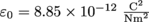. Verify that the electric force still has units of Newtons.

2. Darth Vader’s 2 meter long light saber, which can be approximated as a thin rod of length L with a uniformly distributed positive charge Q, lies on the x-axis as seen below. Yoda stands confidently at a distance b away from the saber, at point P. What electric field does the light saber produce for b = 1 m, left in terms of charge Q?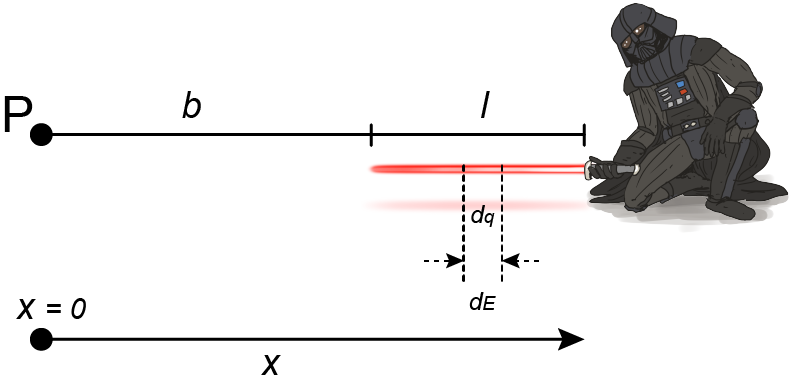3. Yoda parried Darth Vader’s blow from #2, but now he stands at a point on the line of the perpendicular bisector of the light saber. What is the total charge Q, if the point on which he stands is 1 m away and has an electric field of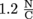?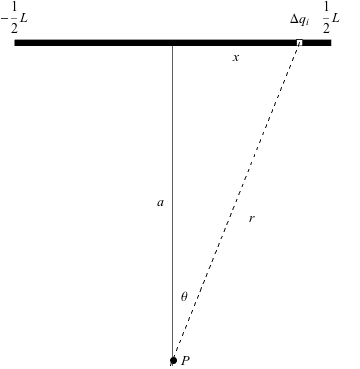4. The main quest in J.R.R. Tolkien’s Lord of the Rings trilogy is to destroy an evil ring forged in the fires of Mount Doom. Let’s say the ring of power has radius a and (evil) charge Q as seen in the diagram below. Low and behold, the dark Lord Sauron rises in the midst of our Shmoop studies. We jump up, on guard at a distance x = 0.4 m from the ring at a point P. What’s the electric field at that position some r = 0.5 m for a charge Q = 80 nC??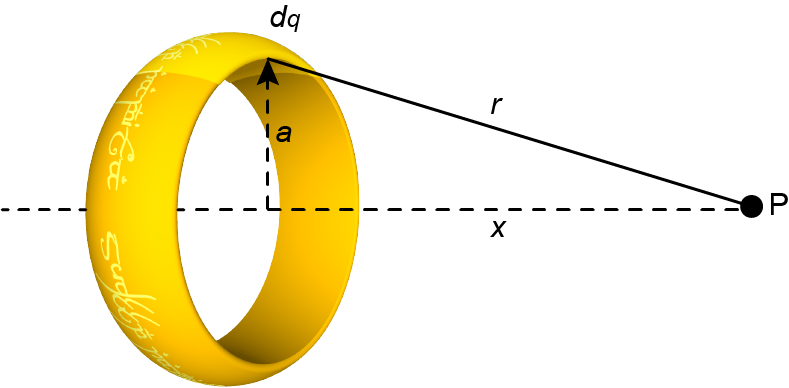5. What is the total electric field right in between two point charges placed 1 m away from each other on the x-axis? The first charge is +10 nC and the other is +4 nC.

1. Answer: Substituting in units for each variable into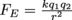, with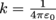produces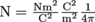. 1/ has no units to worry about.

2. Answer: First, let’s remember that the Force will be with you….always.

The electric field at some point P, a distance b away from, but on axis of a uniformly charged rod is determined by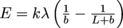. Rather than freaking out that we don’t know a numberic value of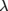, we may use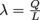. Substituting in all other values, we find that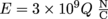. That sounds large, but since a charge is most often recorded in nanoCoulumbs, Yoda may not be in as much danger as the number appears to indicate.

3. Answer: Don’t worry, we’re not the ones confronting Vader…yet. This time we need the equation for a distance off-axis from a charged rod,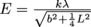where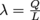. We plug everything in to solve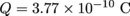or 377 pC. Yoda doesn’t have much to fear at that distance.

4. Answer: Given r, the best equation to choose is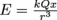. It doesn’t matter that we lack a since we know r and x. Using that information, we calculate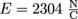. Run away!

5. Answer: The electric field flows positive to negative, always. Since these two charges are both positive, the point 0.5 m away from each will be in the positive direction for one, and the negative direction for the other.

But we’re getting ahead of ourselves: let’s calculate the field from each force from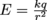for each: for the 10 nC charge, it’s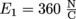and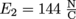in the opposite direction, so the total electric field is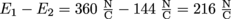.

Consider trying the bonus challenge question of finding the exact distance between the two charges where there is no net electric field.

### Electric Potential and Beyond

1. An electric charge of q = 10 μC is surrounded by a sphere of radius r = 0.5 m. What is the electric flux that goes through this sphere?

2. The main quest in J.R.R. Tolkien’s Lord of the Rings trilogy is to destroy an evil ring forged in the fires of Mount Doom. Let’s say the ring of power has radius a and (evil) charge Q as seen in the diagram below. Low and behold, the dark Lord Sauron rises in the midst of our Shmoop studies. We jump up on your guard at a distance x from the ring at a point P. What’s the electric potential at that position some r = 0.5 m for a charge Q = 80 nC?3. What is the value of the electric field for an infinite charged plane with 2 nC of for every square centimeter?

4. What is the electric dipole moment for a charge of 3.2 pC and a distance of 0.8 pm?

5. What is the torque on the dipole from #4 if it’s placed perpendicularly in the constant electric field between two plates, each 0.5 cm2 with a charge of 4 nC?

1. Answer: The electric field E of a point charge is given by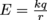, which gives us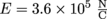.

The flux is given by Φ= EA. Since E is constant, we calculate just the surface area of a sphere with A = 4πr2. Multiplying E with A we then get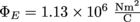.

2. Answer: Since V = Ed where d = r we’ve got to find the field first. For a ring,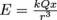. It doesn’t matter that we lack a or x since we know r. Using that information, we calculate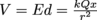= 1152 V. Alternatively, we could take the value we found a while back of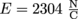and multiply by 0.5 m. Either way, that’s a lot of voltage staring at us in the face.

3. Answer: Infinite charged planes may not be real, but we could still find their hypothetical traits. We learned in two places—the sections on electric fields, and the one about Gauss’ Law—that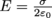, where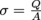. We know the charge in SI units, and the area is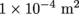. The permittivity constant is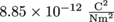, so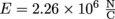.

4. Answer: The electric dipole moment is determined by p = qd, and we already have the values of the charge and distance, so p = 2.56 × 10-24 Cm.

5. Answer: One thing leads to another in this problem. We do know the dipole moment from #4 is p = 2.56 × 10-24 C m.Torque is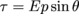, but sin (90) = 1, so we’re left with τ = Ep. Great, now we need the electric field for two charged plates. That’s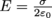. And now we need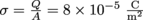. At last we can stop chasing down numbers and work back to the torque. First,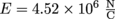. Lastly, τ = 1.16 × 10-17 N m.

### Magnetic Fields

1. Find the magnetic field B at center point P of a current carrying loop of radius r = 4 cm with a steady current I = 2 A.

2. A solenoid with 3 inches of 300 turns per inch has a magnetic field within of 20 μT. What’s the current?

3. Let’s say a wire has a length L of 5.0 m and carries a current of 25 A that flows from left to right on the x-axis. A magnetic field B at an angle of 45° from the x-axis with a magnitude of 1.5 T. What force does the wire experience? Include direction.

4. Describe the force experienced by two parallel wires with identical currents traveling in the positive x-axis direction, with their displacement from each other in the y-direction.

5. What is the magnetic field 1 cm from a single current carrying wire of I = 0.72 A?

1. Answer: Biot-Savart Law tells us that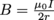for the center of a loop of a current-carrying loop of wire, where the permeability constant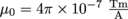, so B = 3.14 × 10-5 T.

2. Answer: Extra information alert: we don’t need the total length of the solenoid to calculate the field inside. The equation for the magnetic field within a solenoid is B = μ0nI, where n is the total number of turns per length, but we have a problem: the length isn’t in standard units. In British units, n = 300 in-1, but in metric, it’s n = 118 cm-1 (turns per centimeter) = 1.18 × 104 m-1.

Anyways, after we substitute everything in we find the current I = 13.5 μA. Or 1.35 × 10-5 A. Gotta love the versatility of our answers in E&M.

3. Answer: First we calculate the magnitude of the force, then use the right hand rule to figure out its direction. Hence we get: F = ILB sin ø = (25 A)(5.0 m)(1.5 T)sin 45°= 133.13 N.

Using the right-hand rule, pointing the index finger along the direction of the current, the fingers in the direction of the magnetic field, means the thumb points in the force direction, which is perpendicular to the horizontal plane of the current and the field. F is along the z-axis, going vertically upwards, out of the computer screen if the x- and y-axis are in the plane of the screen.

4. Answer: We learned in the section about magnetic fields and forces that any two parallel wires with charges traveling in the same direction attract each other. The forces will be towards each other in the y-direction. We can verify this by using the right hand rule of pointing the thumb along the direction of the current (x-direction) to find the direction of each of the magnetic fields at the location of the other wire (z-direction), then use the other right hand rule to find the force in the y-direction towards each other in each case.

5. Answer: We dig up the appropriate equation: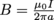, where a is the distance from the wire for which we want to find the field. Therefore, B = 9.05 nT. We sure love these plug-and-chug kinds of problems.

### Magnetic Field Relatives

1. What’s the current density J for a wire with 10 nC per second in an area of 1 square millimeter?

2. What’s the number of charged particles with charge q = 1.6 × 10-19 C for each electron in problem #1 if the drift velocity is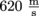?

3. What’s the electrical conductivity of the wire of problem #1 if the electric field is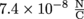?

4. What is the magnetic dipole moment for a current loop with 5 turns of wire, I = 0.64 A, and a radius of 1.3 cm?

5. What is the current within a solenoid with 300 turns over a length of 4 cm if the magnetic potential is 0.6 × 10-6 T m at a radius of 6 mm from the center of the solenoid. Assume that the solenoid has a radius greater than 6 mm.

1. Answer: We know three different equations for current density: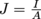J = nqvd, and J = σE,where σ is the electric conductivity of the material, and not the charge per area. We have the ability to calculate the current as 10 nA and then divide it by 1 × 10-6 m2 for a density J of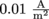.

2. Answer: Oh, goodie—we get to use another version of the current density equation, J = nqvd, with n = 1 and q = 1.6 × 10-19 C, which is the charge of an electron but positive, because that’s how current is defined. We found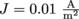in #1. Then we just solve for the number of particles, n, which is 1 × 1014 particles, an unfathomably large number: 100 trillion. Oh, our national debt is almost that large. Not that we can fathom that either.

3. Answer: Earlier we used J = σE to solve for the conductivity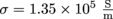. Don’t worry about the units: they’re the standard SI units for conductivity but we didn’t talk about them in the chapter so we’re off the hook.

4. Answer: The equation to determine the magnetic moment is m = NIA. We already have I spelled out for us, the area is A = πr2, and N = 5. All told, m = 1.7 × 10-3 A m2.

5. Answer: The one and only equation for magnetic potential we learned is for just this case, the potential within a solenoid where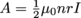. In SI units, r = 0.006 m and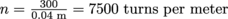.

### Electricity and Magnetism Together at Last

1. If the equation for the electric field of an electromagnetic wave is Ey = 0.03ei(235,619,449y – 50t), what is the frequency?

2. If the equation for the electric field of an electromagnetic wave is Ey = 0.03ei(235,619,449y – 50t), what is the wavelength?

3. If the equation for the electric field of an electromagnetic wave is Ey = 0.03ei(235,619,449y – 50t), what is the equation for the magnetic field?

4. If the equation for the electric field of an electromagnetic wave is Ey = 0.03ei(235,619,449y – 50t), what direction is the wave propagating and how do we know?

5. If the equation for the electric field of an electromagnetic wave is Ey = 0.03ei(235,619,449y – 50t), what direction is the polarization of the electric and magnetic components?

1. Answer: We find ω from the exponent in the function, of the form (kyωt), then convert it to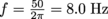.

2. Answer: We could arrive at the answer two ways: we could use the frequency we found in #1 in c = , or we could find the wavelength from the wave number k = 2πλ. Either way, λ = 3.75 × 107 m. Wow, is that a long wave.

3. Answer: They’re related: By = B0ei(235,619,449y – 50t). We don’t know the initial value for the magnetic field, but we know everything else.

4. Answer: It propagates in the direction specified inside the exponent, (kyωt) in this case, so y’s the answer.

5. Answer: The wave propagates in the y-direction, so therefore one of them oscillates in the x-direction, and the other in the z-direction. We can use the pictures in the electromagnetic wave section to figure out that either orientation is acceptable. If, however, the electric field was specified to oscillate in the z-direction, then the magnetic field would have to oscillate in the x-direction, etc.

### Circuits

1. What’s the natural frequency for an LC circuit with an 4 mH inductor and a 90 nC capcitor?

2. Find a combination of inductance and capacitance which yield a natural frequency of 2 Hz. Hint: convert f to w first.

3. A musical physicist added a speaker to an RLC circuit with a natural frequency of 20 Hz, corresponding to the lower limit of human hearing for sound waves. The capacitor in the circuit is 80 μC. What must the resistance in the circuit be for the oscillations to be critically damped (with no sound)?

4. What’s the period of oscillation in a circuit with an inductance of 50 μH, a capacitance of 20 nC and a resistance of 200 Ω?

5. Let’s say we want to build an RLC circuit with a quarter of the un-damped LC frequency. What resistor value should we use?

1. Answer: The equation we need is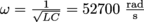.

2. Answer: Well, since ω = 2πf, the target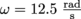. Then we need to find an L and C combination that will produce this value. Really, we can make up any number for one of them and solve for the other. It’s a little easier to do that if we get LC out from under the radical, as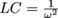= 0.0064. For example, if we choose C = 1 mC, then L = 6.4 H.

3. Answer: The natural frequency allows us to find the inductance from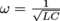. First, convert f = 20 Hz to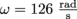, leading to L = 0.79 H. Next, we use the conditions of critical damping by setting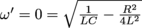, and we solve for R = 3.95 kΩ.

4. Answer: We begin with the equation for the frequency of a damped circuit,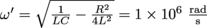. Frequency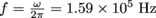. Period is the inverse of frequency,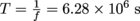, or 6.28 μs.

5. Answer: The frequency of an LC circuit with no resistance is just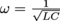, while for an RLC circuit, it’s a tad more complicated with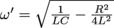. We want ω’ to be a quarter of ω, so all we need to do is plug and chug: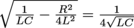. After a lot of algebra beginning with squaring both sides, we find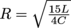.